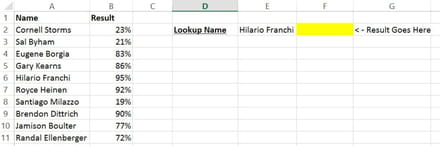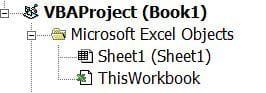# VBA Coding Vlookup to single workbook from different tabs [Closed]

-
Posts
4
Registration date
Thursday June 2, 2016
Status
Member
Last seen
June 2, 2016
-
Hello,

I am trying to pull data through Vlookup to master sheet from different worksheet which is having a different tabs for different client.

I am able to pull the data for 1 client (HSBC) but for different clients. I am not able to do so.
Please help me with th coding.

Sub Macro2()
'
' Macro2 Macro
'
' Keyboard Shortcut: Ctrl+Shift+M
'
Columns("J:J").Select
Selection.Insert Shift:=xlToRight
Range("J2").Select
ActiveCell.FormulaR1C1 = "=VLOOKUP(RC[-1],'[Updated Ppt.xlsx]HSBC'!C9,1,0)"
Selection.AutoFill Destination:=Range("J2:J42")
Range("J2:J42").Select
Range("J1").Select
Selection.AutoFilter
ActiveSheet.Range("\$A\$1:\$N\$42").AutoFilter Field:=10, Criteria1:="#N/A"
End Sub

## 1 reply

Posts
4
Registration date
Thursday June 2, 2016
Status
Member
Last seen
June 2, 2016

Hi Shivani,

You'll find the below article from excelvlookuphelp.com useful

2 (of many) ways to do a vlookup in vba

There are two ways that you might want to use this. Either (1) putting a vlookup in a cell or (2) using a vlookup to return a value in another way such as a message box.

This post assumes a basic knowledge of excel vba and macros.

Here’s an example list of names and results in columns A and B respectively.

(1) We’re going to use VBA to put a vlookup formula into cell F2Press Alt + F11 to get into the VBA editor and right click on the little icon at the top left that will have the text VBAProject with your workbook name in brackets after it, like this:From the menu that appears when you right click, select Insert>> Module. This is where you’ll type your VBA code as follows:

Sub MakeVlookupInCell()

Range(“F2”).Select
ActiveCell.FormulaR1C1 = “=VLOOKUP(RC[-1],C[-5]:C[-4],2,FALSE)”

End Sub

What this is doing is choosing cell F2 and putting in the following criteria for our vlookup formula (see “How to do a vlookup” article)

SearchFor RC[-1] refers to the cell that is one column to the left of the cell that the formula is in (so cell E2)

WhereToSearch C[-5]:C[-4] translates to columns A:B where A is 5 columns to the left of the formula cell and B is 4 columns to the left

WhichColumn the second column in the search table, namely column B.

NearOrExact False makes the vlookup search for an exact match

To run the macro, you can either click somewhere between Sub and End Sub and press F5 or you can go back to the Excel worksheet, press Alt F8 to bring up the list of macros, select your MakeVlookupInCell one and press Run.

(2) Instead of using a vlookup formula, why not do the following:

Follow the steps as above to insert a module if you haven’t already.

Type in the following text:

Sub ResultInMsgBox()

Dim iX As Integer
Dim strSearchString As String

strSearchString = Range(“E2”).Value
iX = 1

Do While Range(“A” & iX).Value <> “”
If Range(“A” & iX).Value = strSearchString Then
MsgBox (strSearchString & “‘s result is ” & (Round(Range(“B” & iX).Value*100, 0) ) & “%”)
Exit Sub
Else
iX = iX + 1
End If
Loop

End Sub

What this does is gets the value in cell E2 and then runs down column A until it finds it. When it does find it, it returns the corresponding value in column B, just like a vlookup would do but in a message box with a bit of text on either side of the result. Not a bad alternative.

Source: http://www.excelvlookuphelp.com/how-do-i-do-a-vlookup-in-vba/